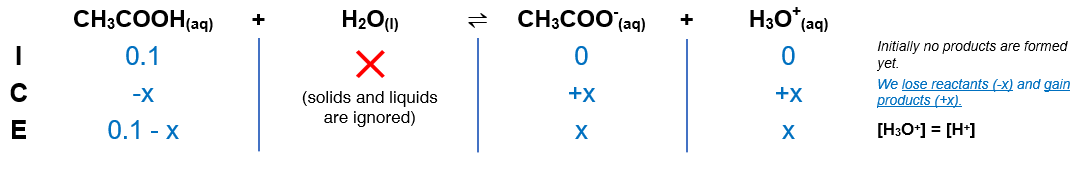# Problem: The Ka of acetic acid (HC2H3O2) is 1.8 x 10-5. What is the pH at 25.0 °C of an aqueous solution that is 0.100 M in acetic acid?a. -2.87b. +11.13c. -11.13d. +6.61e. +2.87

###### FREE Expert Solution

(1) Construct an ICE table(2) Calculate the [H3O+] using Ka expression

82% (417 ratings)###### Problem Details

The Ka of acetic acid (HC2H3O2) is 1.8 x 10-5. What is the pH at 25.0 °C of an aqueous solution that is 0.100 M in acetic acid?

a. -2.87
b. +11.13
c. -11.13
d. +6.61
e. +2.87